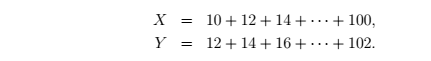How 9 Cheenta students ranked in top 100 in ISI and CMI Entrances?

# Problem Solving Marathon Week 1

Cheenta has planed to initiate a problem solving Marathon with existing students. Here we are providing the problems and hints of "Problem Solving Marathon Week 1". The Set comprises three levels of questions as following-Level 0- for Class III-V; Level 1- for Class VI-VIII; Level 2- for the class IX-XII. You can post your alternative idea/solution in here.

## Level 0

(Q.1)Which of the following is equal to${1 + \frac{1}{1+\frac{1}{1+1}}}$${1 + \frac{1}{1+\frac{1}{1+1}}}$?

Hint 1
Calculate${1+\frac{1}{1+1}}$

Hint 2
Try to use the fact${\frac{\frac{a}{b}}{\frac{c}{d}} =\frac{a \times d}{b \times c}}$

(Q.2)Let${X}$${X}$ and${Y}$${Y}$ be the following sums of arithmetic sequences:What is the value of${Y - X}$${Y - X}$?

Hint 1
Observe that how many terms are there, in$X$ and$Y$. If there are$n$ nos. of terms then pair up like$(1^{st}$ term,$n^{th}$ term$)$,$(2^{nd}$ term,${n-1}^{th}$ term$)$

Hint 2
If you add the elements of every pair, then you will get same result of every pair.

## Level 1

(Q.1)Find all positive integers$n$$n$ such that$n^2+1$$n^2+1$ is divisible by$n+1$$n+1$.

Hint 1$n^2+1$ can be written as$n(n+1)-(n-1)$

Hint 2
Try to find the necessary condition for$(n+1)|(n-1)$

(Q.2)Two geometric sequences$a_1, a_2, a_3, \ldots$$a_1, a_2, a_3, \ldots$ and$b_1, b_2, b_3, \ldots$$b_1, b_2, b_3, \ldots$ have the same common ratio, with$a_1 = 27$$a_1 = 27$,$b_1=99$$b_1=99$, and$a_{15}=b_{11}$$a_{15}=b_{11}$. Find$a_9$$a_9$. Example of Geometric Sequence$2,4,8,16$$2,4,8,16$, here common ratio is$2$$2$.

Hint 1
Try to find the$n$th term of geometric sequence

Analyze the example$2,4.8,16$ is an example of geometric sequence. Here common ratio is$2$. Now$1^{st}$ term$=2$.$2^{nd}$ term$=1^{st}$ term$\cdot$$2$,$3^{rd}$ term$=1^{st}$ term$\cdot$$2^2$,$4^{th}$ term$=1^{st}$ term$\cdot$$2^3$.

## Level 2

(Q.1)Let$m$$m$,$n$$n$,$p$$p$ be real numbers such that$m^2 + n^2 + p^2 - 2mnp = 1$$m^2 + n^2 + p^2 - 2mnp = 1$. Prove that$(1+m)(1+n)(1+p) \leq 4 + 4mnp$$(1+m)(1+n)(1+p) \leq 4 + 4mnp$

Hint 1
Note that$(m+n+p)^2 = m^2 + n^2 + p^2 + 2(mn+np+pmx)$

Hint 2
Here you can use the idea of Cauchy Schwarz Inequality

# Knowledge Partner